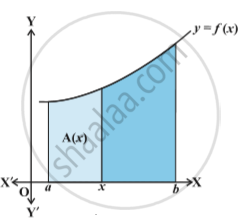# Fundamental Theorem of Calculus

#### description

Area function, First fundamental theorem of integral calculus and Second fundamental theorem of integral calculus

#### notes

Area function:int_a^b f(x) dx as the area of the region bounded by the curve y = f(x), the ordinates x = a and x = b and x-axis. Let x be a given point in [a, b]. Then int_a^x f(x) dx represents the area of the light shaded region in above fig.   [Here it is assumed that f(x) > 0 for x ∈ [a, b], the assertion made below is equally true for other functions as well]. The area of this shaded region depends upon the value of x.
In other words, the area of this shaded region is a function of x. We denote this function of x by A(x). We call the function A(x) as Area function and is given by
A(x) = int_a^x f(x) dx    ....(1)
Based on this definition, the two basic fundamental theorems have been given.

1) First fundamental theorem of integral calculus:
Theorem:  Let f be a continuous function on the closed interval [a, b] and let A (x) be the area function. Then A′(x) = f (x), for all x ∈ [a, b].

2) Second fundamental theorem of integral calculus:
Theorem: Let f  be continuous function defined on the closed interval [a, b] and F be an anti derivative of f. Then int_a^b f(x) dx = [F(x)]_a^b = F(b) -F(a)

Remarks:
(i) In words, the Theorem 2 tells us that ∫_a^b f(x) dx = (value of the anti derivative F of f at the upper limit b – value of the same anti derivative at the lower limit a).

(ii) This theorem is very useful, because it gives us a method of calculating the definite integral more easily, without calculating the limit of a sum.

(iii) The crucial operation in evaluating a definite integral is that of finding a function whose derivative is equal to the integrand. This strengthens the relationship between differentiation and integration.

(iv) In ∫_a^b f(x) dx , the function f needs to be well defined and continuous in [a, b].

Steps for calculating int_a^b f(x) dx

(i) Find the indefinite integral ∫ f(x) dx . Let this be F(x). There is no need to keep integration constant C because if we consider F(x) + C instead of F(x), we get
∫_a^b f(x) dx = [F(x) + C]_a^b = [F(b) + C] - [F(a) + C] - [F(a) + C] =F(b)-F(a) . Thus, the arbitrary constant disappears in evaluating the value of the definite integral.

(ii) Evaluate F(b) – F(a) = [F(x)]_a^b , which is the value of ∫_a^b f(x) dx .

If you would like to contribute notes or other learning material, please submit them using the button below.

#### Video Tutorials

We have provided more than 1 series of video tutorials for some topics to help you get a better understanding of the topic.

Series 1

Series 2

### Shaalaa.com

Fundamental Theorem of Calculus [00:11:17]
S
0%